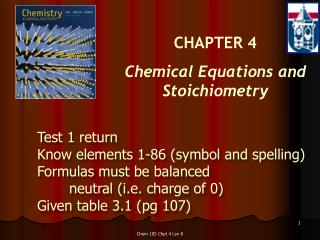DownloadDownload PresentationCHAPTER 4 Chemical Equations and Stoichiometry

# CHAPTER 4 Chemical Equations and Stoichiometry

Download Presentation## CHAPTER 4 Chemical Equations and Stoichiometry

- - - - - - - - - - - - - - - - - - - - - - - - - - - E N D - - - - - - - - - - - - - - - - - - - - - - - - - - -
##### Presentation Transcript

1. CHAPTER 4 Chemical Equations and Stoichiometry Test 1 return Know elements 1-86 (symbol and spelling) Formulas must be balanced neutral (i.e. charge of 0) Given table 3.1 (pg 107) Chem 105 Chpt 4 Lsn 8

2. Balanced equations(from neutral formulas) • Conservation of matter (Lavoisier) • Stoichiometry (stoichiometric coefficients) • Relationship between quantities prod. & react • 5 step process (pg 146) • Always start with neutral formulas Chem 105 Chpt 4 Lsn 8

3. Conservation of matter (Lavoisier) Chem 105 Chpt 4 Lsn 8 p.169b

4. Balancing equations • Aluminum and bromine combine to produce aluminum bromide. This is reacted with sulfuric acid (H2SO4). Hydrogen bromide (HBr) and aluminum sulfate are produced. 1.) Write the formulas for the reactants and products. 2.) Balance the entire reaction. 3.) Verify an equal number of atoms on reactant and product side. Chem 105 Chpt 4 Lsn 8

5. Chem 105 Chpt 4 Lsn 8

6. Balancing an ionic equation • Number of atoms and charge must be balanced. • Aluminum oxide (Al2O3) and monatomic hydrogen gas combine to form water and aluminum ion. • Write formulas for balanced reactants and products. • Balance the reaction. • Double check. • Al2O3 + 6 H+ 2 Al3+ + 3 H2O Chem 105 Chpt 4 Lsn 8

7. Balancing with a polyatomic ion • Remember to keep the ion together • Phosphoric acid (H3PO4) and barium hydroxide combine to form barium phosphate and water. • Balance the starting material formulas and then the equation • H3PO4 + Ba(OH)2 Ba3(PO4)2 + H2O • 2H3PO4 +3 Ba(OH)2 Ba3(PO4)2 +6 H2O Chem 105 Chpt 4 Lsn 8

8. Mass relationships (use stoichiometric relation) Chem 105 Chpt 4 Lsn 8

9. Mass relationships Na2SO4 (aq)+ BaCl2 (aq)  NaCl (aq) + BaSO4 (s) Chem 105 Chpt 4 Lsn 8 Fig. 4.8, p.159

10. Mass relationships Na2SO4 (aq)+ BaCl2 (aq)  NaCl (aq) + BaSO4(s) • Balance the equation • How many moles of NaCl will be produced from 0.81 moles of Na2SO4? • How many grams of NaCl will be produced from 0.81 moles of Na2SO4? • How many grams of BaSO4 will be produced from 3.76 grams of BaCl2? Chem 105 Chpt 4 Lsn 8 p.149

11. Limiting reactant (reagent) • Which reactant is limiting? • How much of product can be formed? • Ammonia gas can be prepared by the reaction of a metal oxide such as calcium oxide with ammonium chloride. If 112g of CaO and 224g of NH4Cl are mixed, what mass of NH3 can be produced? Chem 105 Chpt 4 Lsn 8

12. Limiting reactant (reagent) • 24. Aluminum chloride, AlCl3, is made by treating scrap aluminum with chlorine. 2 Al (s) + 3 Cl2 (g)  2 AlCl3 (s) If you begin with 2.70 g of Al & 4.05 g of Cl2 a) Which reactant is limiting? b) What mass of AlCl3 can be produced? c) What mass of the excess reactant remains after the hexane has been burned? Chem 105 Chpt 4 Lsn 8

13. Next Lesson • Percent yield • Chemical equations and chemical analysis Chem 105 Chpt 4 Lsn 8

14. Chem 105 Chpt 4 Lsn 8 p.162b

15. Balancing Equations ____C3H8(g) + _____ O2(g) ----> _____CO2(g) + _____ H2O(g) ____B4H10(g) + _____ O2(g) ----> ___ B2O3(g) + _____ H2O(g) Chem 105 Chpt 4 Lsn 8